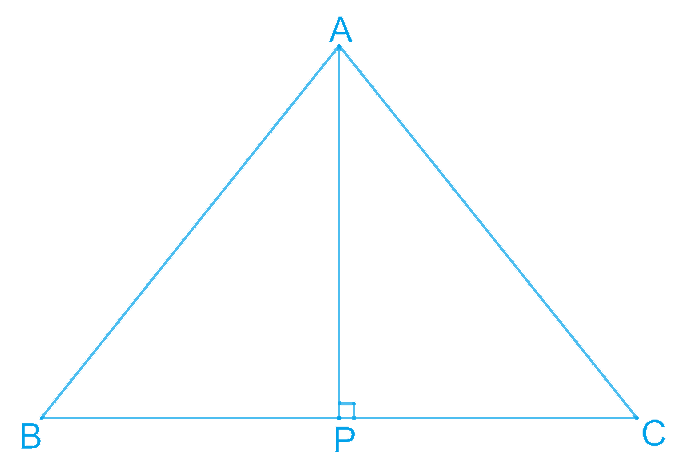# Ex.7.3 Q5 Triangles Solution - NCERT Maths Class 9

Go back to  'Ex.7.3'

## Question

$$ABC$$ is an isosceles triangle with

$$AB = AC$$. Draw $$AP ⊥ BC$$ to show that $$\angle B = \angle C$$.

Video Solution
Triangles
Ex 7.3 | Question 5

## Text Solution

What is Known?

$$ABC$$ is an isosceles triangle with $$AB \;\;AC$$ and $$AP \;BC$$

To prove:

$\angle \text{B}=\angle \text{C}$

Reasoning:

We can show triangles $$APB$$ and $$APC$$ congruent by using RHS congruency and then we can say corresponding parts of congruent triangles are equal to show the required result.

Steps:In $$\Delta APB$$ and $$\Delta APC$$,

\begin{align} \angle APB & = \angle APC\;(\text{Each }90^{\circ})\\ AB &= AC \;(\text {Given})\\ AP& = AP \;(\text {Common})\\ \Delta APB &\cong \Delta APC \\&(\text {Using RHS congruence rule})\end{align}

$\angle B = \angle C\;(\text{By using }CPCT)$

Video Solution
Triangles
Ex 7.3 | Question 5

Learn from the best math teachers and top your exams

• Live one on one classroom and doubt clearing
• Practice worksheets in and after class for conceptual clarity
• Personalized curriculum to keep up with school